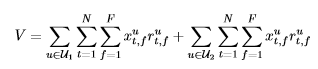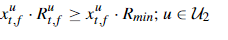•Gurobi Staff

Could you post a minimal working example of what you are currently trying to implement?

How to write this constraint when a variable belongs to a set.

Depending on how you define your variable in your code, you can define a list of indices and loop over those, e.g.,

import gurobipy as gpm=gp.Model()x = m.addVars(10)U = [0,1,2,3]m.addConstrs((x[u] <= 2) for u in U)

constructs the constraints $$x_u \leq 2 \quad u \in U = \{0,1,2,3\}$$.

Best regards,
Jaromił

•Hi Jaromil,

Thanks for the reply. Here is the problem I am trying to solve:

U_1 = {1,2,3,..L}

U_2 = {1,2,3,..K}

R=r^{u}_{t,f} is the cost function. I have to maximize the above objective function using constraints C1,C2, and C3 in the above problem I have posted before. Here is the code I wrote:

T = (np.arange(1,21,1))
#print(len(T))
F = (np.arange(1,101,1))
M = np.arange(1,11,1)
E = (np.arange(1,6,1))
U = (np.arange(1,6,1))

assignment_model = grb.Model('Assignment')

x =  assignment_model.addVars(M.shape, T.shape, F.shape, vtype = grb.GRB.CONTINUOUS, lb = 0, ub = 1,name = 'x')
assignment_model.addConstrs((sum(x[u, t, f]  for u in range(E.shape) for u in range(U.shape)) <= 1 for t in range(T.shape) for f in range(F.shape)), name = 'one RB allocation')
assignment_model.addConstrs((sum(x[u,t,f] for t in range(T.shape) for f in range(F.shape)) >= 1 for u in range(U.shape) ), name = 'latency requirement')
obj_fun = sum(se_e_power[p][u,t,f] * x[u,t,f] for u in range(E.shape) for t in range(T.shape) for f in range(F.shape)) + sum(se_u_power[p][u,t,f] * x[u,t,f] for u in range(U.shape) for t in range(T.shape) for f in range(F.shape))
assignment_model.setObjective(obj_fun, grb.GRB.MAXIMIZE)
assignment_model.setParam('OutputFlag', False)
assignment_model.optimize()
#print('Optimization is done. Objective function value: %.2f' % assignment_model.objVal)
value = assignment_model.objVal

It looks like I wrote the third constraint as you suggested. But the output is not as expected. Please let me know if there is anything wrong with code.

Thanks

•Gurobi Staff

Hi Venkateswarlu,

The constraint

assignment_model.addConstrs((sum(x[u,t,f] for t in range(T.shape) for f in range(F.shape)) >= 1 for u in range(U.shape) ), name = 'latency requirement')

Looks OK. Did you write the model to an LP file and inspect it to see whether all constraints look as expected?

assignment_model.write("myLP.lp")

The write method will write a human-readable LP file which you can inspect.

Best regards,
Jaromił

•Hi Jaromil,

I am using the write command, but I do not see any output. I updated the version to latests but I do not see any output.

Any suggestions?

Thanks,

Venkat

•Gurobi Staff

Hi Venkat,

The write method will generate a file in the folder from which you execute your script. It will not print anything to the console output or the log.

Best regards,
Jaromił

•I can see the output now

•Hi,

I guess I solved the issue after printing it. Thanks for the suggestions.

One more question is what kind of algorthim the libraray is using. As my problem is convex optimization, I was looking into theorteical concept of solving the problem. It was explained there are many algorthims to solve it. What exactly the algorthim it uses when I write grb. Maximize(like sub-gradient or Newtons or lagrangian). Is there any documentation which explains the algaorthim the library is using?

Thanks,

Venkat

•Gurobi Staff

Hi Venkat,

You can find some basics on convex continuous programming at Linear Programming (LP) - A Primer on the Basics. There at the bottom, you will find the literature for the algorithms you seek.

Best regards,
Jaromił

•Hi Jaromil,

Thanks for the information.

I have a quick one. I am trying to write the following constraint but I am getting an error. Could you suggest me how to write the following expression? t,f are for all. R_min is a constant.